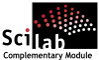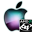ATOMS : Assert details

# Assert

A collection of predicate functionsDetails
Version
1.0
A more recent valid version with binaries for Scilab 5.2 exists: 1.3
Author
Michael Baudin
Owner Organization
DIGITEO
Maintainers
Michael BAUDIN
Allan CORNET
Categories
Creation Date
October 4, 2010
Source created on
Scilab 5.2.x
Binaries available on
Scilab 5.2.x:Windows 64-bitWindows 32-bitLinux 64-bitLinux 32-bitmacOS
Install command
`--> atomsInstall("assert")`
Report a bugDescription
```            Purpose
----

The goal of this toolbox is to provide functions to make testing easier.
We emphasize the numerical issues, with the goal of testing numerical algorithms
more
easily. In particular, we provide a comparison function for two floating
point numbers, which allows to assert that two numbers are "close"
(including, but not limited to, equal).

The function of the assert module are designed to be used in Scilab unit tests
files (.tst files).

The following is a list of the current features:

* assert for true, equal statements
* assert that two floating point numbers are "close". Use an relative or a
absolute tolerance
* provide a sorting function for double complex numbers with a mixed
relative/absolute tolerance criteria.

The following is a list of the current assert functions :
* assert_checkalmostequal : Check that computed and expected are numerically
close.
* assert_checkequal : Check that computed and expected are equal.
* assert_checktrue : Check that condition is true.
* assert_checkfalse : Check that condition is false.
* assert_checkfilesequal : Check two files are equal.
* assert_checkerror : Check that the function has produced the correct error.
* assert_generror : Generates an error.
and sorting functions :
* assert_compare : The default comparison function used in the sort-merge.
* assert_comparecomplex : Compare complex numbers with a tolerance.
* assert_sort : A flexible sorting function.
* assert_sortcomplex : Compare complex numbers with a tolerance.
and support functions :
* assert_benchfun : Benchmarks a function and measure its performance.
* assert_computedigits : Returns the number of significant digits in computed
result.
* assert_condition2reltol : Suggests a relative error, computed from the
condition number.
* assert_expandvar : Expand variables so that they all have the same shape.
* assert_printtable : Prints a table of data with a given number of digits.
and condition number functions :
* assert_acoscond : Computes the condition number of the acos function.
* assert_asincond : Computes the condition number of the asin function.
* assert_atancond : Computes the condition number of the atan function.
* assert_condnum : Computes the empirical condition number of the function f at
point x.
* assert_coscond : Computes the condition number of the cos function.
* assert_erfcond : Computes the condition number of the erf function.
* assert_erfinvcond : Computes the condition number of the erfinv function.
* assert_expcond : Computes the condition number of the exp function.
* assert_logcond : Computes the condition number of the log function.
* assert_plotcond : Plots the condition number of a function.
* assert_powcond : Computes the condition number of the pow function.
* assert_sincond : Computes the condition number of the sin function.
* assert_sqrtcond : Computes the condition number of the square root function.
* assert_sumcond : Computes the condition number of the sum function.
* assert_tancond : Computes the condition number of the tan function.

The toolbox is based on macros.

To get an overview of the functions in this module,
use :

help assert_overview

TODO
----

* TODO : create assert_datasetread, assert_datasetwrite for dataset management
* TODO : create assert_computerelativeerror with management for nan, inf
* TODO : create assert_checkdigits
* TODO : create assert_condmaxsearch, based on a combination of fminsearch and
assert_condnum

```Files (2)Source code archive
```
```OS-independent binary for Scilab 5.2.x
```Binary version
Automatically generated by the ATOMS compilation chain

```News (0)Comments (0)Leave a comment
Login withEmail notifications